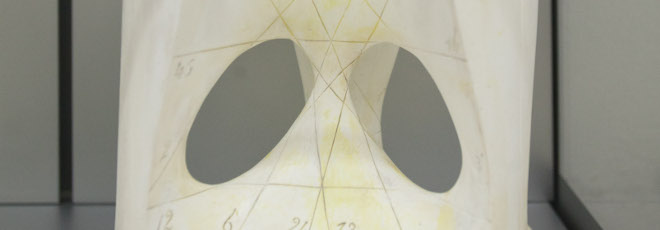## “Gradient of the single layer potential and rectifiability”

Venerdì 24 Gennaio 2020, ore 11:00 - Aula 430 - Carmelo Puliatti (Universitat Autònoma de Barcelona)

Abstract

An important theorem by Nazarov, Tolsa and Volberg asserts that a measure $\mu$ on $\mathbb{R}^{n+1}$ which has $n$-polynomial growth both from above and from below (also known as $n$-Ahlfors-David regular measure) and whose associated $n$-Riesz transform is bounded on $L^2(\mu)$ is $n$-uniformly rectifiable.

In this talk I will discuss an analogous result for the operator \begin{equation*} T_\mu f(x)=\int \nabla_x \mathcal{E}_A(x,y)f(y)d\mu(y), \end{equation*} where $A$ is an $(n+1)\times(n+1)$-uniformly elliptic matrix with Hölder continuous coefficients and $\mathcal{E}_A(\cdot,\cdot)$ is the fundamental solution to the equation \begin{equation*} L_A u \equiv -\text{div}(A(\cdot)\nabla u)=0. \end{equation*}

This is a joint work with Laura Prat and Xavier Tolsa, and it is also motivated by an application to the study of elliptic measure.

I will also present a recent closely related article which contains a quantitative rectifiability criterion for more general Radon measures in terms of $T_\mu$. This applies to a two-phase problem for the elliptic measure.